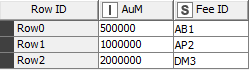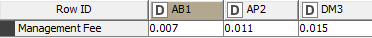# Conditional calculation (math formula)

Dear community,

i started using KNIME and I stumbled into a problem.

Table A which consists of 2 columns: feeID and amount.There is table B, which contains feeIDs and percentage amount of discount.What I want to do is multiply amount from table A with discount from table B based on feeID.

I tried to use math formula but i can not access any string column there.

There are more complex cases but this is the basic one.

Any ideas? What approach to use?
(I know that I can transpose the table but how should I do conditional calculation?)

Thank you upfront.
Jure

I think what you want to try here is the Column Expressions node, since it can handle conditionals, string manipulation, and math calculation all in the same node.

Here’s an example workflow to give you an idea:

Note that Column Expressions will require installing an additional extension, but you will be prompted to do this when you open the workflow.

2 Likes

Dear ScottF,

thank you for your feedback.

But I don’t see how Column Expression is helping me with this problem since I have data in 2 tables.
Are you suggesting that I should move this data from table B and hard code it in this node?

Tables connected with Joiner node if they have common key.

1 Like

I implemented join, but if you imagine table with 100k rows, adding additional x columns (which are constants) does not sound like optimal solution?

As Igor suggested, I was assuming you would do some sort of Join beforehand. Example workflow attached - I did this without Column Expressions. I’m not adding a ton of columns since there’s a transpose first. So I think it should be relatively efficient…?

TransposeMathFormulaExample.knwf (14.6 KB)

1 Like

This topic was automatically closed 182 days after the last reply. New replies are no longer allowed.